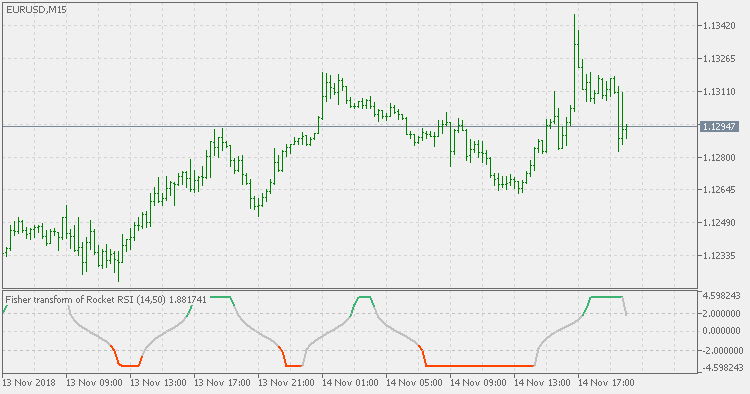Interesting script?
So post a link to it -
let others appraise it
You liked the script? Try it in the MetaTrader 5 terminal# Rocket RSI - indicator for MetaTrader 5

|
Views:
7720
Rating:
Published:
2018.11.14 19:19
Updated:
2019.01.29 16:28

Theory:

In “RocketRSI—A Solid Propellant For Your Rocket Science Trading”, author John Ehlers introduces a new take on the classic RSI indicator originally developed by J. Welles Wilder. Ehlers begins by introducing a new version of the RSI based on a simple accumulation of up and down closes rather than averages. To this he applies a Fisher transform. He tells us that the resultant output is statistically significant spikes that indicate cyclic turning points with precision.

All in all, in the terms that we are used to, it is an RSI of a smoothed momentum (2 pole super smoother used for smoothing the momentum). It might and might not deserve the given name, but it deserves a version that works exactly the same as the original so that we all can test what exactly it does.

This version:

Is calculated the original way, it is producing exactly the same results as the original and has some options added:

• you can chose the price (original uses close only)
• you can chose to calculate Fisher transform of the "rocket" RSI or to see the "rocket" RSI itself
• you have 3 coloring options:
• color changes on slope change
• color changes on zero line cross
• color changes on levels cross (which is what has been used as trigger for entries in the original article)

Usage:

You have to experiment with this indicator to find interesting settings. One is at the example picture (you can see the settings from the picture). In any case, when you set it up, you can use the color change as signal.Range weighted smoothed EMA

Range weighted smoothed EMARange weighted EMA

Range weighted exponential moving average (EMA)Stochastic of filtered price

Stochastic of filtered priceStochastic of average(s)

Stochastic of average(s)